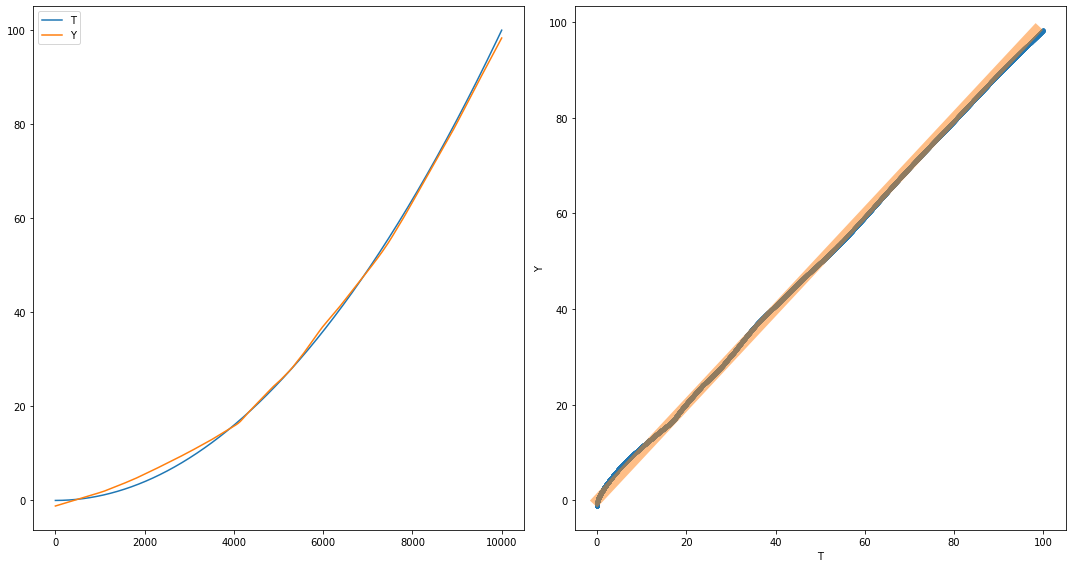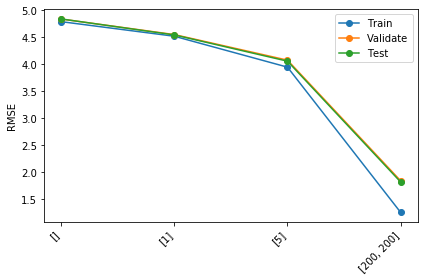# NNet class in Pytorch¶

Versions:

• 3.3: Added calls to np.random.seed(42) to force the shuffle in our partition function to result in the same random arrangement of samples each time, to help with your debugging.
• 3.2: RMSE in example output of generate_k_fold_cross_validation_sets are corrected. A3grader.zip updated.
• 3.1: A3grader.zip is now available. The generate_k_fold_cross_validation_sets function had an error that is fixed in this version.

• 3.0: A3grader.zip is not available yet.

In this assignment you will complete an implementation of a neural network class that uses pytorch. You will then use it to conduct some training experiments on specified data. The data will be partitioned into some number of non-intersecting folds. You will train, validate and test neural networks of various sizes on all ways of picking one fold to be testing, one fold to be validation, and remaining folds to be training sets.

## Implementation¶

In :
import numpy as np
import torch
import pandas
import matplotlib.pyplot as plt


Complete the following definition of class NNet by completing its train function.

In [ ]:
class NNet(torch.nn.Module):

def __init__(self, n_inputs, n_hiddens_per_layer, n_outputs, act_func='tanh'):
super().__init__()  # call parent class (torch.nn.Module) constructor

# Set self.n_hiddens_per_layer to [] if argument is 0, [], or 
if n_hiddens_per_layer == 0 or n_hiddens_per_layer == [] or n_hiddens_per_layer == :
self.n_hiddens_per_layer = []
else:
self.n_hiddens_per_layer = n_hiddens_per_layer

self.hidden_layers = torch.nn.ModuleList()  # necessary for model.to('cuda')

for nh in self.n_hiddens_per_layer:
self.hidden_layers.append( torch.nn.Sequential(
torch.nn.Linear(n_inputs, nh),
torch.nn.Tanh() if act_func == 'tanh' else torch.nn.ReLU()))

n_inputs = nh

self.output_layer = torch.nn.Linear(n_inputs, n_outputs)

self.Xmeans = None
self.Xstds = None
self.Tmeans = None
self.Tstds = None

self.error_trace = []

def forward(self, X):
Y = X
for hidden_layer in self.hidden_layers:
Y = hidden_layer(Y)
Y = self.output_layer(Y)
return Y

def train(self, X, T, n_epochs, learning_rate, verbose=True):

# Set data matrices to torch.tensors if not already.
if not isinstance(X, torch.Tensor):
X = torch.from_numpy(X).float()
if not isinstance(T, torch.Tensor):
T = torch.from_numpy(T).float()

# Calculate standardization parameters if not already calculated
if self.Xmeans is None:
self.Xmeans = X.mean(0)
self.Xstds = X.std(0)
self.Xstds[self.Xstds == 0] = 1
self.Tmeans = T.mean(0)
self.Tstds = T.std(0)
self.Tstds[self.Tstds == 0] = 1

# Standardize inputs and targets
X = (X - self.Xmeans) / self.Xstds
T = (T - self.Tmeans) / self.Tstds

# Set optimizer to Adam and loss functions to MSELoss
mse_func = torch.nn.MSELoss()

# For each epoch:
#   Do forward pass to calculate output Y.
#   Calculate mean squared error loss, mse.
#   Calculate gradient of mse with respect to all weights by calling mse.backward().
#   Take weight update step, then zero the gradient values.
#   Unstandardize the mse error and save in self.error_trace
#   Print epoch+1 and unstandardized error if verbose is True and
#             (epoch+1 is n_epochs or epoch+1 % (n_epochs // 10) == 0)

for epoch in range(n_epochs):

. . .

def use(self, X):

# Set input matrix to torch.tensors if not already.
if not isinstance(X, torch.Tensor):
X = torch.from_numpy(X).float()

# Standardize X
. . .

# Do forward pass and unstandardize resulting output. Assign to variable Y.
. . .

# Return output Y after detaching from computation graph and converting to numpy
return Y.detach().numpy()


## Example¶

In :
torch.manual_seed(42)
np.random.seed(42)

n_samples = 10000
X = np.linspace(0, 10, n_samples).reshape((-1, 1))
T = X ** 2

n_samples, n_inputs = X.shape
n_outputs = T.shape

n_hiddens = [100, 100]
net = NNet(n_inputs, n_hiddens, n_outputs, act_func='relu')
net.train(X, T, 50, 0.01)
Y = net.use(X)

def rmse(Y, T):
return np.sqrt(np.mean((T - Y)**2))

print(f'RMSE {rmse(Y, T):.3f}')

Epoch 5: RMSE 11.155
Epoch 10: RMSE 6.062
Epoch 15: RMSE 5.169
Epoch 20: RMSE 2.103
Epoch 25: RMSE 2.317
Epoch 30: RMSE 2.636
Epoch 35: RMSE 1.463
Epoch 40: RMSE 1.137
Epoch 45: RMSE 1.170
Epoch 50: RMSE 0.922
RMSE 0.867

In :
plt.figure(figsize=(15, 8))

plt.subplot(1, 2, 1)
plt.plot(T, label='T')
plt.plot(Y, label='Y')
plt.legend()

plt.subplot(1, 2, 2)
plt.plot(T, Y, '.')
a = max(np.min(T), np.min(Y))
b = min(np.max(T), np.max(Y))
plt.plot([a, b], [a, b], lw=10, alpha=0.5)
plt.xlabel('T')
plt.ylabel('Y')
plt.tight_layout()# Function to generate all train, validate, and test partitions given number of folds¶

## Implementation¶

This is complete code for generate_k_fold_cross_validation_sets.

In :
def generate_k_fold_cross_validation_sets(X, T, n_folds, shuffle=True):

if shuffle:
# Randomly order X and T
randorder = np.arange(X.shape)
np.random.shuffle(randorder)
X = X[randorder, :]
T = T[randorder, :]

# Partition X and T into folds
n_samples = X.shape
n_per_fold = round(n_samples / n_folds)
n_last_fold = n_samples - n_per_fold * (n_folds - 1)

folds = []
start = 0
for foldi in range(n_folds-1):
folds.append( (X[start:start + n_per_fold, :], T[start:start + n_per_fold, :]) )
start += n_per_fold
folds.append( (X[start:, :], T[start:, :]) )

# Yield k(k-1) assignments of Xtrain, Train, Xvalidate, Tvalidate, Xtest, Ttest

for validation_i in range(n_folds):
for test_i in range(n_folds):
if test_i == validation_i:
continue

train_i = np.setdiff1d(range(n_folds), [validation_i, test_i])

Xvalidate, Tvalidate = folds[validation_i]
Xtest, Ttest = folds[test_i]
if len(train_i) > 1:
Xtrain = np.vstack([folds[i] for i in train_i])
Ttrain = np.vstack([folds[i] for i in train_i])
else:
Xtrain, Ttrain = folds[train_i]

yield Xtrain, Ttrain, Xvalidate, Tvalidate, Xtest, Ttest


## Example¶

In :
X = np.arange(2 * 6).reshape((6, 2))
T = np.arange(6).reshape((6, 1)) * 10
X, T

Out:
(array([[ 0,  1],
[ 2,  3],
[ 4,  5],
[ 6,  7],
[ 8,  9],
[10, 11]]),
array([[ 0],
,
,
,
,
]))
In :
np.random.seed(42)

for Xtrain, Ttrain, Xvalidate, Tvalidate, Xtest, Ttest in generate_k_fold_cross_validation_sets(X, T, 3):
print()
print('Xtrain\n', Xtrain)
print('Ttrain\n', Ttrain)
print('Xvalidate\n', Xvalidate)
print('Tvalidate\n', Tvalidate)
print('Xtest\n', Xtest)
print('Ttest\n', Ttest)


Xtrain
[[8 9]
[6 7]]
Ttrain
[
]
Xvalidate
[[0 1]
[2 3]]
Tvalidate
[[ 0]
]
Xtest
[[10 11]
[ 4  5]]
Ttest
[
]

Xtrain
[[10 11]
[ 4  5]]
Ttrain
[
]
Xvalidate
[[0 1]
[2 3]]
Tvalidate
[[ 0]
]
Xtest
[[8 9]
[6 7]]
Ttest
[
]

Xtrain
[[8 9]
[6 7]]
Ttrain
[
]
Xvalidate
[[10 11]
[ 4  5]]
Tvalidate
[
]
Xtest
[[0 1]
[2 3]]
Ttest
[[ 0]
]

Xtrain
[[0 1]
[2 3]]
Ttrain
[[ 0]
]
Xvalidate
[[10 11]
[ 4  5]]
Tvalidate
[
]
Xtest
[[8 9]
[6 7]]
Ttest
[
]

Xtrain
[[10 11]
[ 4  5]]
Ttrain
[
]
Xvalidate
[[8 9]
[6 7]]
Tvalidate
[
]
Xtest
[[0 1]
[2 3]]
Ttest
[[ 0]
]

Xtrain
[[0 1]
[2 3]]
Ttrain
[[ 0]
]
Xvalidate
[[8 9]
[6 7]]
Tvalidate
[
]
Xtest
[[10 11]
[ 4  5]]
Ttest
[
]


# Function to train NNets and average RMSE over all ways of partitioning¶

## Implementation¶

Define the following function that trains neural networks of different architectures, given by list_of_n_hiddens, for one value each of n_epochs, learning_rate, and act_fun. For each network, train on all k-fold cross-validation training sets, and calculate the RMSEs for the training, validation and test. Calculate the mean of training, validation and test RMSEs over the k-fold partitions, so that you have one training, one validation, and one test set RMSE for each network. Append a list of the architecture and these three mean RMSEs to a list of results and return the list as a pandas DataFrame described below when done.

To iterate over all k-fold partitions, use a for loop like the example in the previous code cell. Call generate_k_fold_cross_validation_sets with shuffle=True.

In [ ]:
def run_k_fold_cross_validation(X, T, n_folds, list_of_n_hiddens,
n_epochs, learning_rate, act_func):

. . .


## Apply to Airfoil Data¶

Download airfoil_self_noise.dat from Airfoil Data Website and read it as shown below.

In :
airfoil = pandas.read_csv('airfoil_self_noise.dat', delimiter='\t',
names=('frequency', 'angle', 'chord',
'velocity', 'thickness', 'pressure'))


Apply your run_k_fold_cross_validation to the airfoil data, with X being the first five columns of the data and T being the last column, the 'pressure'.

Here is an example run. You must try more neural network architectures. Keep the following points in mind when you choose parameter values to try:

• Larger networks will require more epochs of training to converge.
• Large learning rates could limit convergence, but small learning rates may take many more epochs than you have patience for.
• Display results as a pandas DataFrame and in plots. Your tabular results and plots must be described with at least 10 sentences.
• Name the columns of your DataFrame result to be columns=('Arch', 'Train RMSE', 'Validate RMSE', 'Test RMSE'))
In :
X.shape, T.shape

Out:
((1503, 5), (1503, 1))
In :
torch.manual_seed(42)
np.random.seed(42)

import time
start = time.time()

results = run_k_fold_cross_validation(X, T, 5,
[[], , , [200, 200]],
200, 0.01, 'tanh')

elapsed = (time.time() - start) / 60/ 60
print(f'Took {elapsed:.2f} hours')
results

Took 0.01 hours

Out:
Arch Train RMSE Validate RMSE Test RMSE
0 [] 4.790511 4.839218 4.840742
1  4.519531 4.550374 4.544003
2  3.945287 4.075278 4.056469
3 [200, 200] 1.247426 1.835065 1.811737

Here is a handy function to plot the RMSEs you have in your results table. You may use this function to plot your results. Remember to describe what you see.

In :
def plot_results(results):
errors = results.iloc[:, 1:].values
archs = results.iloc[:, 0].values
archs = [str(ar) for ar in archs]

plt.plot(archs, errors, 'o-')
plt.legend(('Train', 'Validate', 'Test'))
plt.ylabel('RMSE')
plt.xticks(range(errors.shape), archs, rotation=45, ha='right')
plt.tight_layout()

In :
plot_results(results)Your notebook will be partially run and graded automatically. Test this grading process by first downloading A3grader.zip and extract A3grader.py from it. Run the code in the following cell to demonstrate an example grading session. You should see a perfect execution score of 90/90 if your functions are defined correctly. The remaining 10 points will be based on other testing and the results you obtain and your discussions.

A different, but similar, grading script will be used to grade your checked-in notebook. It will include additional tests. You should design and perform additional tests on all of your functions to be sure they run correctly before checking in your notebook.

For the grading script to run correctly, you must first name this notebook as Lastname-A3.ipynb| with Lastname being your last name, and then save this notebook and check it in at the A3 assignment link in our Canvas web page.

In :
%run -i A3grader.py

======================= Code Execution =======================

Extracting python code from notebook named 'instructor-A3.ipynb' and storing in notebookcode.py
Removing all statements that are not function or class defs or import statements.

Testing
torch.manual_seed(142)

X = np.arange(40).reshape(20, 2)
T = np.log(1 + X[:, 0:1])

nnet = NNet(X.shape, [10, 10], T.shape, act_func='relu')
nnet.train(X, T, 100, 0.01, verbose=False)

first_Y = nnet.use(X)[0, 0]

--- 30/30 points. Returned correct value.

Testing
torch.manual_seed(142)

X = np.arange(40).reshape(20, 2)
T = np.log(1 + X[:, 0:1])

nnet = NNet(X.shape, [10, 10], T.shape, act_func='tanh')  # Using tanh
nnet.train(X, T, 100, 0.01, verbose=False)

first_Y = nnet.use(X)[0, 0]

--- 30/30 points. Returned correct value.

Testing
torch.manual_seed(400)
np.random.seed(400)

X = np.arange(40).reshape(20, 2)
T = np.log(1 + X[:, 0:1])

results = run_k_fold_cross_validation(X, T, 3,
[[], , , [40, 40]],
1000, 0.01, 'tanh')

print(results)

# This will take a minute or two....

Arch  Train RMSE  Validate RMSE  Test RMSE
0        []    0.286660       0.476284   0.476284
1           0.111919       0.402824   0.407797
2          0.005308       0.301842   0.294546
3  [40, 40]    0.001703       0.315544   0.316573

--- 30/30 points. Returned correct value.

======================================================================
tmp Execution Grade is 90 / 90
======================================================================

__ / 10 Based on other testing and the results you obtain and your discussions.

======================================================================
tmp FINAL GRADE is  _  / 100
======================================================================

Extra Credit:

1. Add a keyword argument gpu that is False by default. If set to True, move data and
neural network model to the GPU and train and test it there. Compare total execution
time without and with the use of the GPU.

2. Find another data set, not used previously in an assignment or in lecture, and repeat
your training experiment without and with GPU on this new data.

tmp EXTRA CREDIT is 0 / 2


# Extra Credit¶

Earn up to 2 extra credit points on this assignment.

1. Add a keyword argument gpu that is False by default. If set to True, move data and neural network model to the GPU and train and test it there. Compare total execution time without and with the use of the GPU.
2. Find another data set, not used previously in an assignment or in lecture, and repeat your training experiment without and with GPU on this new data.

Describe all results.